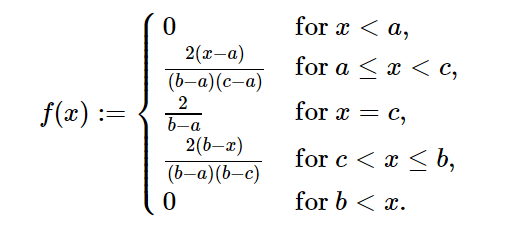# sympy.stats.Triangular() in Python

• Last Updated : 08 Jun, 2020

With the help of `sympy.stats.Triangular()` method, we can get the continuous random variable which represents the triangular distribution.Attention geek! Strengthen your foundations with the Python Programming Foundation Course and learn the basics.

To begin with, your interview preparations Enhance your Data Structures concepts with the Python DS Course. And to begin with your Machine Learning Journey, join the Machine Learning - Basic Level Course

Syntax : `sympy.stats.Triangular(name, a, b, c)`
Where, a, b and c are real number.

Return : Return the continuous random variable.

Example #1 :
In this example we can see that by using `sympy.stats.Triangular()` method, we are able to get the continuous random variable representing Triangular distribution by using this method.

 `# Import sympy and Triangular``from` `sympy.stats ``import` `Triangular, density``from` `sympy ``import` `Symbol, pprint`` ` `z ``=` `Symbol(``"z"``)``a ``=` `Symbol(``"a"``, positive ``=` `True``)``b ``=` `Symbol(``"b"``, positive ``=` `True``)``c ``=` `Symbol(``"c"``, positive ``=` `True``)`` ` `# Using sympy.stats.Triangular() method``X ``=` `Triangular(``"x"``, a, b, c)``gfg ``=` `density(X)(z)`` ` `pprint(gfg)`

Output :

/ -2*a + 2*z
|—————– for And(a z)
|(-a + b)*(-a + c)
|
| 2
| —— for c = z
= z, c < z)
|(-a + b)*(b – c)
|
\ 0 otherwise

Example #2 :

 `# Import sympy and Triangular``from` `sympy.stats ``import` `Triangular, density``from` `sympy ``import` `Symbol, pprint`` ` `z ``=` `5``a ``=` `1.2``b ``=` `1.3``c ``=` `1.27`` ` `# Using sympy.stats.Triangular() method``X ``=` `Triangular(``"x"``, a, b, c)``gfg ``=` `density(X)(z)`` ` `pprint(gfg)`

Output :

0

My Personal Notes arrow_drop_up